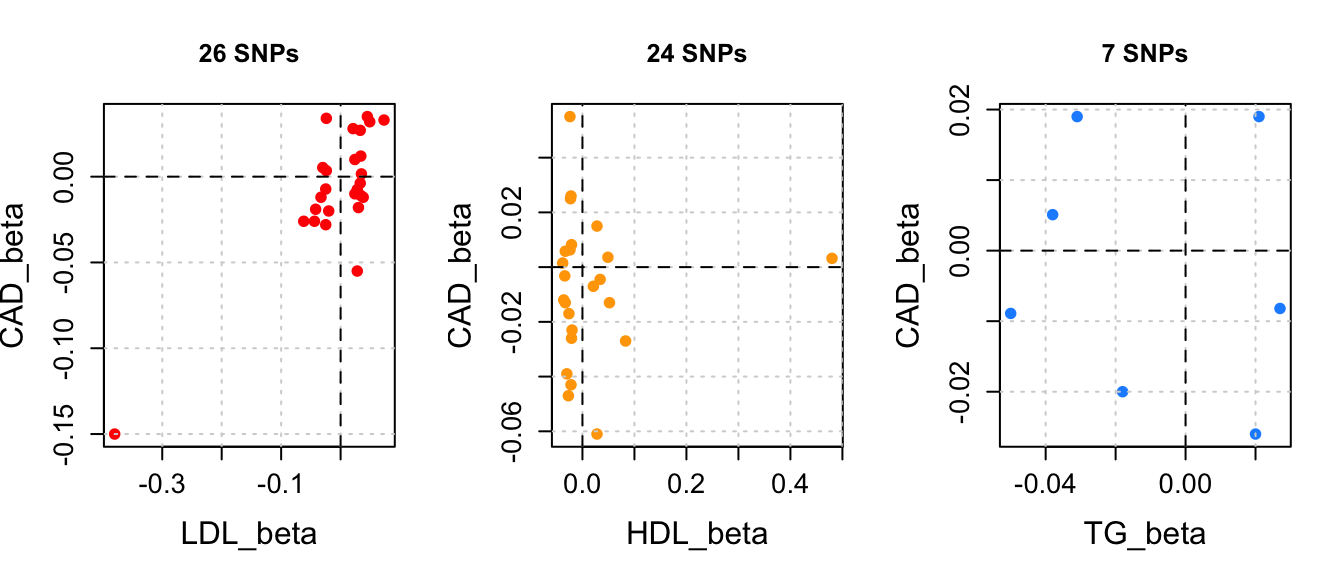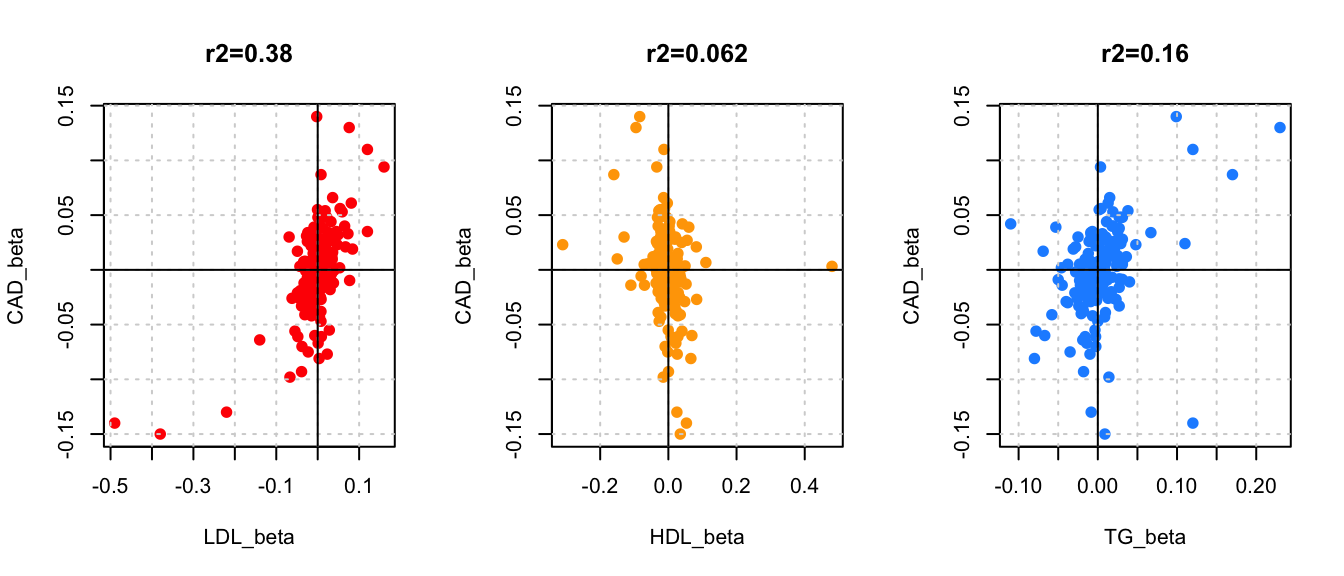The slide set referred to in this document is “GWAS 11”.

Mendelian randomization uses genetic variants to study whether a particular exposure is a causal risk factor for a disease. See slides 2-? A soft introduction by G.Taubes. A review by Davey Smith & Hemani 2014.

Let’s consider the example from Do et al. 2013 Nat Gen. They studied the relationship between LDL-C, HDL-C and triglyserides (TG) on the risk of coronary artery disease (CAD). They collected 185 SNPs that were associated with some of the risk factors (LDL, HDL or TG, or several of them).

The idea in MR is to see how SNPs associated some risk factor of a disease are associated with the disease. If the risk factor has a causal effect on the disease, then the SNPs affecting the risk factor should also affect the disease. Furthermore, we could have a quantitative expectation how much each SNP increases the risk based on how much the SNP changes the risk factor, and how much the risk factor affects the disease risk.

as.is = T, header = T)
x[1,]
##         rsid chr      pos A1 A2 LDL_beta LDL_p HDL_beta HDL_p TG_beta
## 1 rs10903129   1 25641524  A  G   -0.033 4e-19   -9e-04  0.79  -0.008
## 1 0.017   -0.012  0.38 0.4654298
nrow(x) #how many SNPs?
##  185

So we have 185 SNPs, and for each we know its allele1’s effect on HDL, LDL and TG as well as effect on risk for CAD.

Suppose we want to know whether LDL has a causal effect on CAD. In standard MR we would look at SNPs that affect ONLY LDL, and we would study whether they also affect CAD.

#Pick SNPs that affect only one trait, and see whether they affect CAD
p.aff = 5e-8 #P-value < this means SNP is affecting
p.not.aff = 1e-2 #P-value > this means SNP is not affecting
cols = c("red","orange","dodgerblue")
traits = c("TG","LDL","HDL")
par(mfrow=c(1,3))
for(set in 1:3){
foc = traits[1 + set %% 3] #which trait is the focal one, which are side ones
s1 = traits[1 + (set+1) %% 3]
s2 = traits[1 + (set+2) %% 3]

ind = (x[,paste0(foc,"_p")] < p.aff &
x[,paste0(s1,"_p")] > p.not.aff &
x[,paste0(s2,"_p")] > p.not.aff)

xlab = paste0(foc,"_beta"), ylab = "CAD_beta", pch = 19, col = cols[set],
main = paste(sum(ind),"SNPs"), cex.axis = 1.3, cex.lab = 1.5)
grid()
abline(h=0,lty = 2)
abline(v=0,lty = 2)
}The need to remove all SNPs with effects on several traits limits information from 185 to only a handful of SNPs. Let’s do as Do et al. (2013) and use all the SNPs and regress the CAD effects on the effects on traits.

First regression for one trait at a time:

res = data.frame(matrix(NA, ncol=5, nrow=3))
par(mfrow=c(1,3))
for(set in 1:3){
foc=c("LDL","HDL","TG")[set] #which trait is the focal one, which are side ones
y.lab = "CAD_beta"; x.lab = paste0(foc,"_beta")
y = x[,y.lab]; z = x[,x.lab]
lm.fit = lm( y ~ z )
plot(z, y, main = paste0("r2=", signif( cor(z,y)^2, 2)),
xlab = x.lab, ylab = y.lab, col = cols[set], pch = 19)
grid()
abline(h = 0)
abline(v = 0)
res[set,] = c(foc, signif(summary(lm.fit)$coeff[2,],3)) }res ## X1 X2 X3 X4 X5 ## 1 LDL 0.411 0.0388 10.6 9.71e-21 ## 2 HDL -0.178 0.0514 -3.47 0.000659 ## 3 TG 0.444 0.0753 5.9 1.73e-08 All three traits have a significant correlation with CAD effect size. What happens when all three are in the same model ? lm.fit=lm(CAD_beta ~ HDL_beta + LDL_beta + TG_beta, data = x) summary(lm.fit)$coeff[-1,]
##             Estimate Std. Error    t value     Pr(>|t|)
## HDL_beta -0.03707842 0.03896405 -0.9516059 3.425659e-01
## LDL_beta  0.39760797 0.03448943 11.5284005 2.068948e-23
## TG_beta   0.40363936 0.05973513  6.7571516 1.862574e-10

HDL is not important predictor when LDL and TG are in the model. So HDL is not likely to be causal. But LDL and TG might be. However, if we had measured also some other correlated lipids or other biomarkers then we might find a more precise cause for the association between our current LDL and TG effects and CAD effects. For example, ApoB has been suggested as a possible factor behind both LDL-C and TG effects on CAD by Ference et al. 2019.

We cannot ever prove causality using only MR, but we can learn about the stregth of evidence for the causality hypothesis.